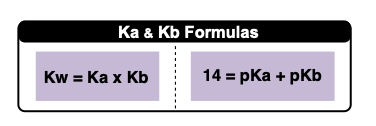Clutch Prep is now a part of Pearson
Ch.15 - Acid and Base EquilibriumWorksheetSee all chapters

# Ka and Kb

See all sections
Sections
Acids Introduction
Bases Introduction
Binary Acids
Oxyacids
Bases
Amphoteric Species
Arrhenius Acids and Bases
Bronsted-Lowry Acids and Bases
Lewis Acids and Bases
The pH Scale
Auto-Ionization
Ka and Kb
pH of Strong Acids and Bases
Ionic Salts
pH of Weak Acids
pH of Weak Bases
Diprotic Acids and Bases
Diprotic Acids and Bases Calculations
Triprotic Acids and Bases
Triprotic Acids and Bases Calculations
Conjugate Acids and Bases

Ka and Kb are the equilibrium constants related to weak acids and weak bases respectively.

###### Ka and Kb constants

Concept #1: The magnitudes of Ka and Kb determine the strengths of acids and bases.

Example #1: Identify the strongest acid from the following list of weak acids based on their Ka values. Assume temp is 25ºC.

a) HCNKa = 4.9 x 10-10

b) H2O Ka = 1.0 x 10-14

c) HNO2 Ka = 4.6 x 10-4

d) HC3H5O3 Ka = 1.4 x 10-4

Practice: Hypobromous acid (Ka = 2.8 × 109) and hydrocyanic acid (Ka = 4.9 × 1010) are both weak acids. Determine if reactants or products are favored in the following reaction.

HBrO (aq) + CN (aq) ⇌ BrO (aq) + HCN (aq)

a) reactants        b) products            c) both directions are favored equally   d) neither direction is favored

Practice: Identify a Bronsted-Lowry acid with weakest conjugate base.

a) H3BO3  Ka = 5.4 × 1010

b) HF Ka = 3.5 × 104

c) HNO2  Ka = 4.6 × 104

d) HClO Ka = 2.9 × 108

Concept #2: The ionization constant of water connects Ka and KbExample #2: Aspirin, also known as acetylsalicylic acid (Ka = 3.3 x 10-4), is a medication used to reduce pain, fever, and inflammation. Calculate the Kb of acetylsalicylate (C9H7O4-).

Practice: Identify which of the compounds is the strongest species.

a) Iodic acid pKa = 0.80  b) Acetic acid pKb = 9.24 c) Formic acid pKa = 3.75 d) Ammonium pKb = 4.75

Practice: Determine the pKa given the Kb of the following bases:

i) NH3               Kb = 1.76 × 105 ; NH4+           pKa = ____________

ii) C6H5NH2     Kb = 3.9 × 1010 ; C6H5NH3+  pKa = ____________